# Work, power & energy Questions and Answers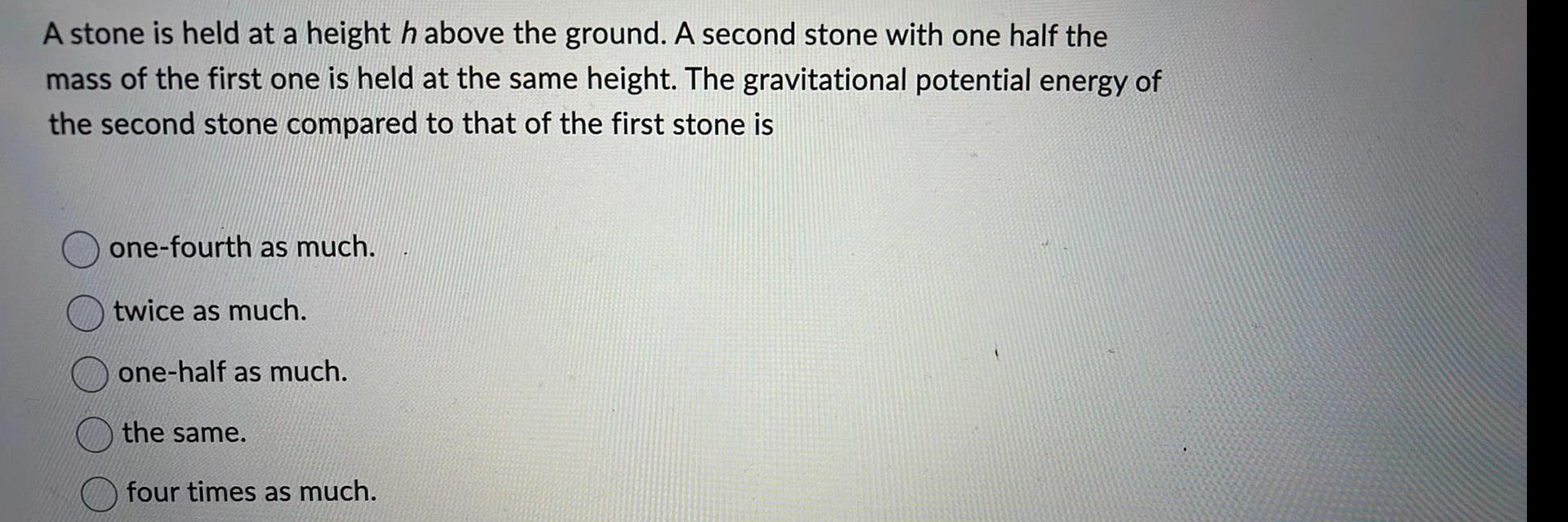Physics
Work, power & energy
A stone is held at a height h above the ground. A second stone with one half the mass of the first one is held at the same height. The gravitational potential energy of the second stone compared to that of the first stone is one-fourth as much. twice as much. one-half as much. the same. four times as much.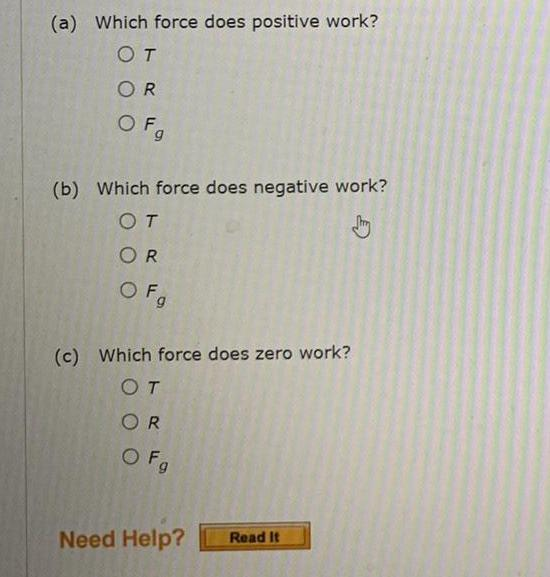Physics
Work, power & energy
(a) Which force does positive work? T R Fg (b) Which force does negative work? T R Fg (c) Which force does zero work? T R Fg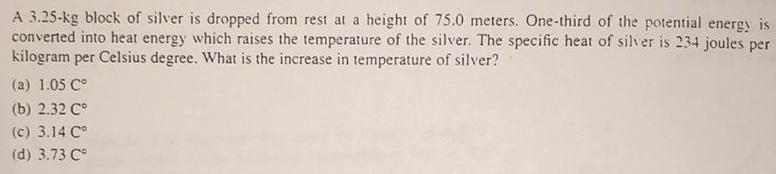Physics
Work, power & energy
A 3.25-kg block of silver is dropped from rest at a height of 75.0 meters. One-third of the potential energy is converted into heat energy which raises the temperature of the silver. The specific heat of silver is 234 joules per kilogram per Celsius degree. What is the increase in temperature of silver? (a) 1.05 C° (b) 2.32 C° (c) 3.14 Cº (d) 3.73 Cº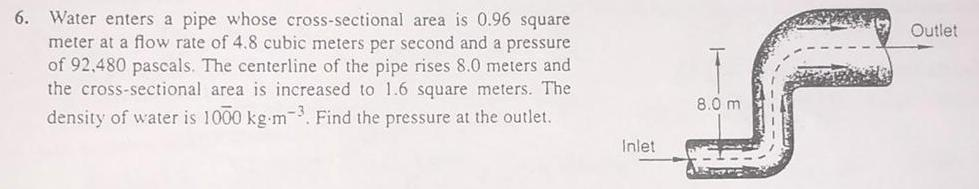Physics
Work, power & energy
Water enters a pipe whose cross-sectional area is 0.96 square meter at a flow rate of 4.8 cubic meters per second and a pressure. of 92,480 pascals. The centerline of the pipe rises 8.0 meters and the cross-sectional area is increased to 1.6 square meters. The density of water is 1000 kg m-³. Find the pressure at the outlet.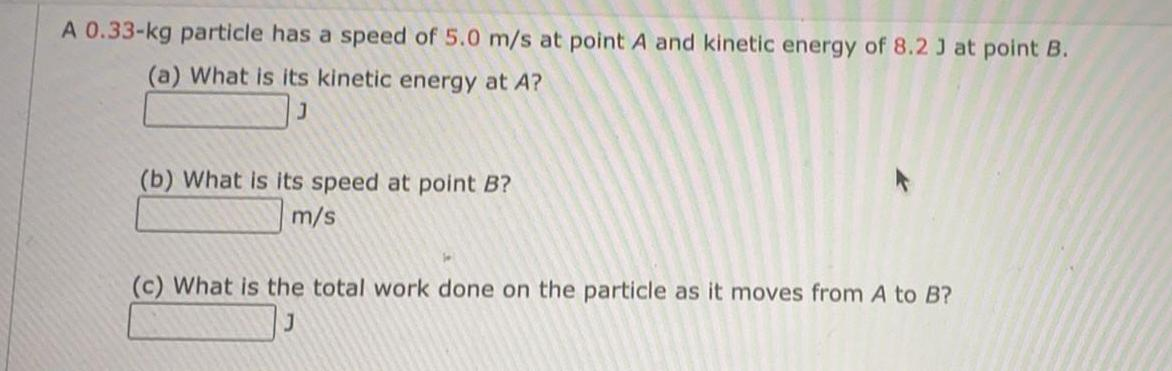Physics
Work, power & energy
A 0.33-kg particle has a speed of 5.0 m/s at point A and kinetic energy of 8.2 J at point B. (a) What is its kinetic energy at A? (b) What is its speed at point B? (c) What is the total work done on the particle as it moves from A to B?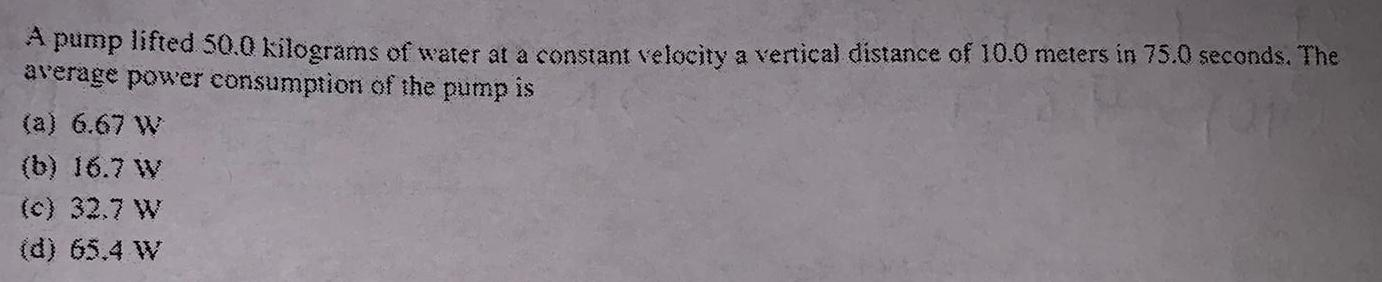Physics
Work, power & energy
pump lifted 50.0 kilograms of water at a constant velocity a vertical distance of 10.0 meters in 75.0 seconds. The average power consumption of the pump is (a) 6.67 W (b) 16.7 W (c) 32.7 W (d) 65.4 W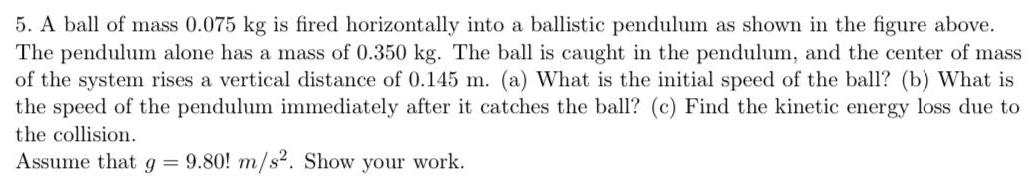Physics
Work, power & energy
A ball of mass 0.075 kg is fired horizontally into a ballistic pendulum as shown in the figure above. The pendulum alone has a mass of 0.350 kg. The ball is caught in the pendulum, and the center of mass of the system rises a vertical distance of 0.145 m. (a) What is the initial speed of the ball? (b) What is the speed of the pendulum immediately after it catches the ball? (c) Find the kinetic energy loss due to the collision. Assume that g = 9.80! m/s². Show your work.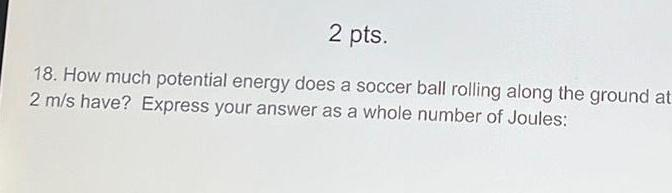Physics
Work, power & energy
How much potential energy does a soccer ball rolling along the ground at 2 m/s have? Express your answer as a whole number of Joules: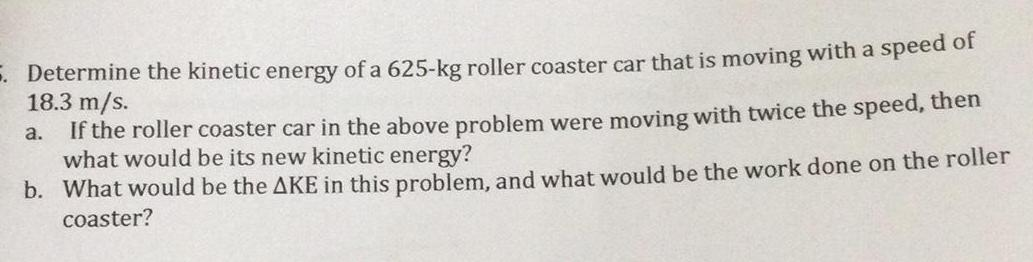Physics
Work, power & energy
Determine the kinetic energy of a 625-kg roller coaster car that is moving with a speed of 18.3 m/s. a. If the roller coaster car in the above problem were moving with twice the speed, then what would be its new kinetic energy? b. What would be the ΔKE in this problem, and what would be the work done on the roller coaster?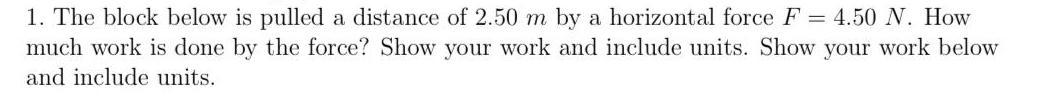Physics
Work, power & energy
The block below is pulled a distance of 2.50 m by a horizontal force F = 4.50 N. How much work is done by the force? Show your work and include units. Show your work below and include units.Physics
Work, power & energy
3. Two objects of different mass start from rest, are pulled by the same net force (magnitude and direction), and are moved through the same displacement. The work done on object 1 is 500 J. After the force has pulled each object, object 1 moves twice as fast as object 2. Answer the following questions. Show your work below and include units. (a) How much work is done on the object 2? (b) What is the kinetic energy of object 1 after being pulled? (c) What is the kinetic energy of object 2 after being pulled? (d) What is the ratio of the mass of object 1 to the mass of object 2?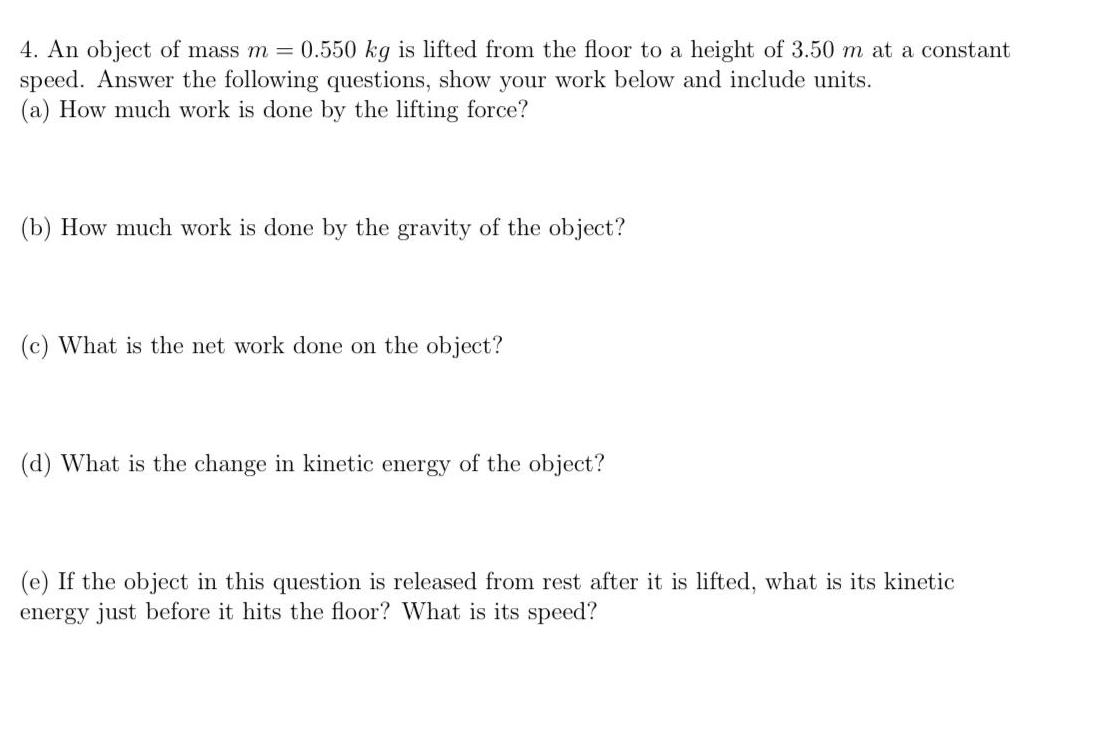Physics
Work, power & energy
An object of mass m = 0.550 kg is lifted from the floor to a height of 3.50 m at a constant speed. Answer the following questions, show your work below and include units. (a) How much work is done by the lifting force? (b) How much work is done by the gravity of the object? (c) What is the net work done on the object? (d) What is the change in kinet energy the object? (e) If the object in this question is released from rest after it is lifted, what is its kinetic energy just before it hits the floor? What is its speed?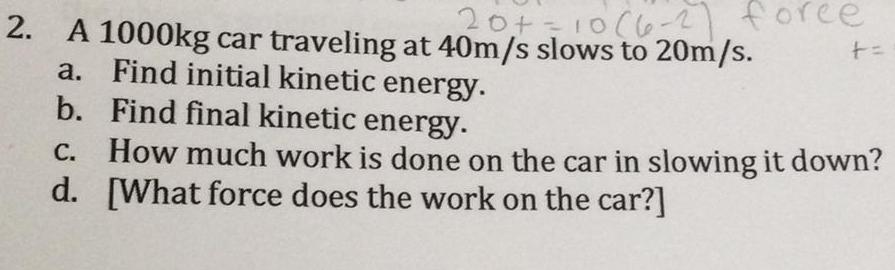Physics
Work, power & energy
2. A 1000kg car traveling at 40m/s slows to 20m/s. a. Find initial kinetic energy. b. Find final kinetic energy. c. How much work is done on the car in slowing it down? d. [What force does the work on the car?]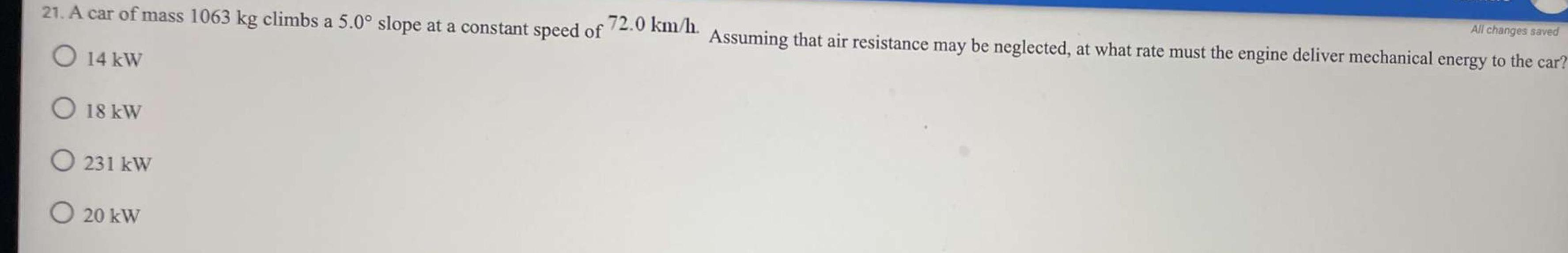Physics
Work, power & energy
A car of mass 1063 kg climbs a 5.0° slope at a constant speed of 72.0 km/h. Assuming that air resistance may be neglected, at what rate must the engine deliver mechanical energy to the car? 14 kW 18 kW 231 kW 20 kW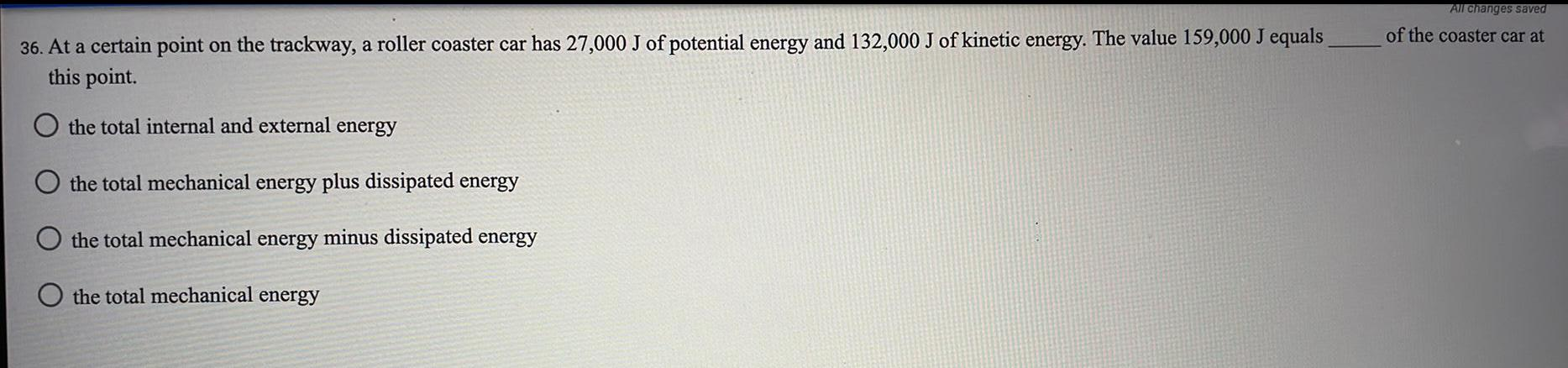Physics
Work, power & energy
At a certain point on the trackway, a roller coaster car has 27,000 J of potential energy and 132,000 J of kinetic energy. The value 159,000 J equals this point. the total internal and external energy the total mechanical energy plus dissipated energy the total mechanical energy minus dissipated energy the total mechanical energy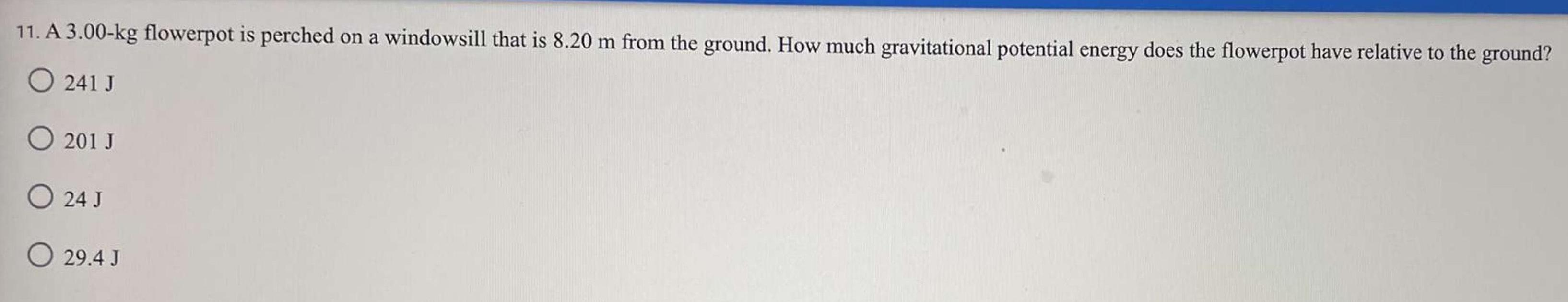Physics
Work, power & energy
A 3.00-kg flowerpot is perched on a windowsill that is 8.20 m from the ground. How much gravitational potential energy does the flowerpot have relative to the ground? 241 J 201 J 24 J 29.4 J